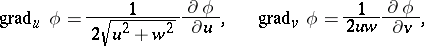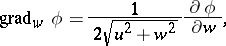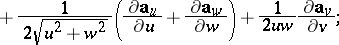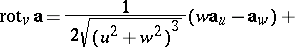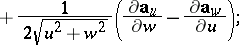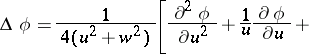# Paraboloidal coordinates

(diff) ← Older revision | Latest revision (diff) | Newer revision → (diff)

Numbers,and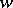related to the rectangular Cartesian coordinates,andby the formulaswhere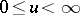,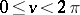,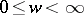. The coordinate surfaces are two systems of paraboloids of revolution with oppositely-directed axes (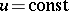and) and half-planes (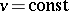). The system of paraboloidal coordinates is orthogonal.

The Lamé coefficients (or scale factors) are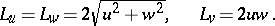The element of surface area is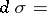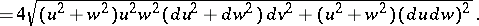The volume element isThe fundamental operations of vector analysis are